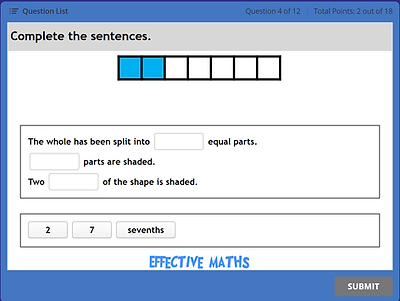top of page

### READY TO PROGRESS QUIZZES - YEAR 3

Number and place value

 3NPV–1

Equivalence of 10 tens and 1 hundred (3NPV–1)

 3NPV–2 - NEW

Place value in three-digit numbers

 3NPV-3 - Coming soon

Three-digit numbers in the linear number system

 3NPV-4 - NEW

Number facts

 3NF–1

[b] Making the previous ten

 3NF–2

[a] Multiplication facts: 4, 8 and 3 × tables

[b] Division facts (4, 8 and 3 × tables)

 3NF–3

Scaling number facts by 10

 3AS–1

Number bonds for 100 (3AS–1)

 3AS–2

[a] Add three-digit numbers (column method) (3AS–2)

[b] Subtract three-digit numbers (3AS–2)

 3AS–3

Inverse relationship and commutative property

Multiplication and division

 3MD–1

Multiplication and division structures

Fractions

 3F–1

 Use and understand fraction notation

 3F–2

Find unit fractions of quantities

 3F-3 - NEW

 3F-4 - NEW

Add and subtract fractions within onebottom of page